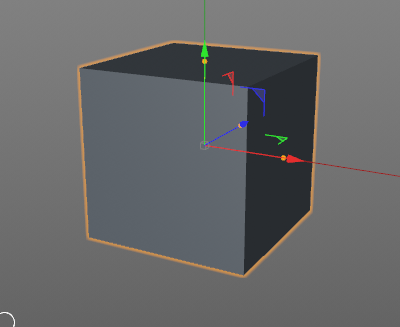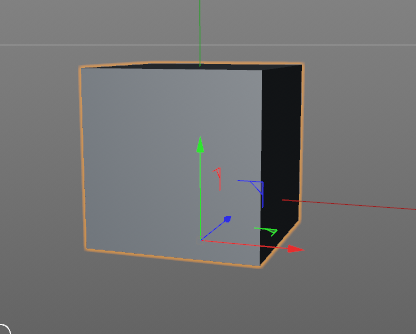# Beginner:How to set axis to the bottom of a cube by python?

• Hi~i am a green hand, well, I think many beginners will meet the problem to set the axis to the specific point, make cube as an easy example, I am wondering how to achieve it``````import c4d
#Welcome to the world of Python

def main():
cube=c4d.BaseObject(c4d.Ocube)

'''how to set axis?(for exaple,to the bottom):grinning: '''

doc.InsertObject(cube)

``````

primitives' axis cannot be changed because their geometry is parametric and generated by the object itself. If the object doesn't come with the function to change the axis, there's no chance to change it via code either.

However, if you have polygon objects, you simply move all points in a desired direction.
For example: If your cube is 200cm in Y dimension and the axis is centered, you simply add Y = 200/2 = 100cm to all points. Then you move the axis/object into the opposite direction for the same value to compensate the position.

• Hi iLad, thanks for reaching out us.

• Matrix Fundamentals: a few basic information on matrices and geometry are provided
• c4d.Matrix: the section describing the matrix class implementation in Cinema 4D
• c4d.PointObject: the section describing the PointObject implementation (the class responsible for handling the vertex data of a generic object)

Wrapping all the above information together, you might end up with something like:

``````import c4d
from c4d import gui
#Welcome to the world of Python

def main():
if op == None:
return

#check about op being derived from a PointObject
if op.IsInstanceOf(c4d.Opoint) == False:
return

# set the offset of the axis
offset = c4d.Vector(100,0,0)

# move object
m = op.GetMg()
m.off = m.off + offset
op.SetMg(m)

# transform points

point = point - offset
op.SetPoint(i,point)

op.Message(c4d.MSG_UPDATE)

if __name__=='__main__':
main()

``````

Note that the above example works properly only if you're supposed to make on offset of the axis and not if you're attempting to change axis orientation: in this latter case you've to compute the inverse transformation matrix and use this to reposition the object in space upon vertexes repositioning.

Best, Riccardo

• @r_gigante Thanks,great help,i will retry in your way later.

• @mp5gosu Thanks,great thought while only limit to still object rather than animated one with botton set axis.• @ilad Well, it's not limited to non-animated objects. As @r_gigante posted, you're actually modifying the points and the matrix. Both operations can also be achieved manually. So, no difference if animated or not.

The only thing you have to keep in mind when modifying animated objects is to make sure, all keys containing Matrix/PLA data have to be handled as well.